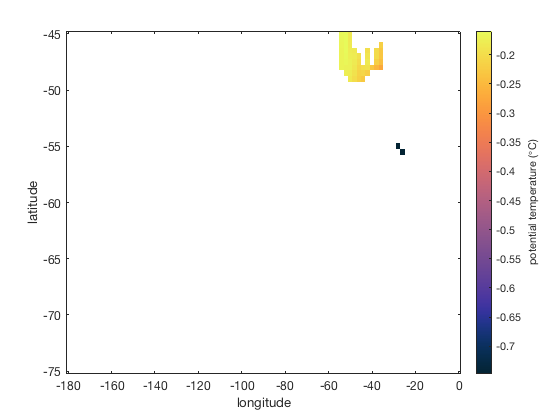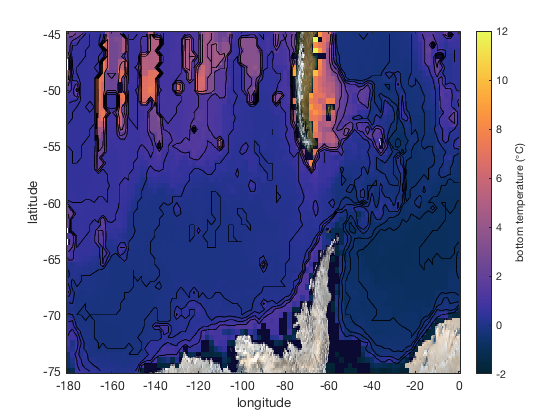# bottom documentation

bottom returns the lowermost finite values in a 3D matrix. This function is useful for getting the seafloor temperature or atmospheric surface temperature in the presence of topography.

## Syntax

```Zb = bottom(Z)
[Zb,ind] = bottom(Z)```

## Description

Zb = bottom(Z) returns a 2D matrix containing the lowermost finite values in the 3D matrix Z.

[Zb,ind] = bottom(Z) also returns 2D matrix ind which contains the indices along the third dimension of Z corresponding to the lowermost finite values in Z.

## Example: Drake Passage

This example uses some gridded ocean temperature data from the Southern Ocean Database (described below). Begin by loading the example data:

```load sodb_example

whos % displays the names and sizes of variables
```
```  Name         Size                 Bytes  Class     Attributes

d           44x1                    352  double
lat          1x61                   488  double
lon          1x91                   728  double
ptm         61x91x44            1953952  double
readme       1x79                   158  char

```

The 61x91x44 matrix ptm contains potential temperatures at latitudes lat, longitudes lon, and depths d. Here's the potential temperature at the deepest depth, d(44)

```imagescn(lon,lat,ptm(:,:,44))

cmocean thermal
cb = colorbar;
ylabel(cb,'potential temperature (\circC)')
xlabel 'longitude'
ylabel 'latitude'
```That's not much information! That's because throughout most of the domain the seafloor is shallower than d(44) = 5500 m deep, so we just get NaN values wherever the the 5500 m layer is deeper than the bathymetry.

So instead, plot the lowermost finite values with the bottom function:

```figure
imagescn(lon,lat,bottom(ptm))

cmocean thermal
caxis([-2 12])
cb = colorbar;
ylabel(cb,'bottom temperature (\circC)')
xlabel 'longitude'
ylabel 'latitude'
```Since we have a depth array d and indices ind corresponding to the deepest finite values at each grid cell, we can get a 2D grid of bottom depths simply from d(ind). Add them to the map as contours:

```% Get bottom temperature and corresponding indices:
[T_bot,ind] = bottom(ptm);

% Make a 2D bottom depth matrix:
D = d(ind);

% Overlay bathymetry contours:
hold on
contour(lon,lat,D,0:1000:5500,'k')

% Underlay a silly-looking earth image:
h = earthimage;
uistack(h,'bottom');
```On a mildly interesting note, the bottom temperature seems to have some relationship to the bottom depth. Compare the two in profile like this:

```figure
plot(T_bot(:),D(:),'.')
axis tight ij % flips y direction
box off
xlabel 'bottom temperature \circC'
ylabel 'bottom depth (m)'
```## How the sodb_example data were acquired

The sodb_example.mat data come from the Southern Ocean Database and were saved using Antarctic Mapping Tools for Matlab (Greene et al., 2017) via the following code:

```lon = -180:2:0;
lat = -75:0.5:-45;
[Lon,Lat] = meshgrid(lon,lat);
[ptm,d] = sodb_interp('ptm',Lat,Lon);
readme = 'potential temperature data from the Southern Ocean Database (Orsi and Whitorth)';